Courses

Test: Inverters- 2

10 Questions MCQ Test Power Electronics | Test: Inverters- 2

Description
Attempt Test: Inverters- 2 | 10 questions in 45 minutes | Mock test for Electrical Engineering (EE) preparation | Free important questions MCQ to study Power Electronics for Electrical Engineering (EE) Exam | Download free PDF with solutions
QUESTION: 1

Match List-I (Type of Inverter circuits) with List-II (Applications/Limitations) and select the correct answer using the codes given below the lists: List-I A. Adjustable voltage inverter B. Pulse width modulated inverter C. Current source inverter D. Voltage source inverter List-II 1. Limited speed range of motor 2. Capable of regeneration 3. Good input power factor at al! frequencies 4. Possibility of short circuit across the source Codes: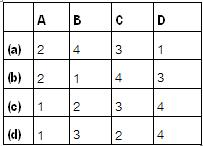Solution:

• By using adjustable voltage inverter, speed range of motor is limited because of motor cogging at 6 Hz and below.
• In pulse width modulated inverter, input p.f. is good at all frequencies.
• A CSI has the capability of regeneration back to the a.c. line because d.c. link polarity can be reversed.
• In VSI, the misfiring of switching devices may cause short circuit across the source and create serious problems.

QUESTION: 2

In current source inverters load voltage waveform V0 and load current waveform i0 respectively

Solution:

In a CSI, load current rather than load voltage is controlled, and the inverter output voltage is dependent upon the load impedance and the output voltage waveform. The load current and hence its waveform is independent of load impedance due to which a CSI has inherent protection against short-circuit across its terminals.

QUESTION: 3

A single phase IGBT bridge inverter, compared to a single pulse PWM control, multiple pulse PWM

Solution:

In case of singte-putse width modulation (PWM), the width of the pulse is adjusted to reduce the harmonic. However, a single phase IGBT bridge inverter produces a square wave. This square wave contains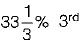harmonic,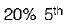harmonic and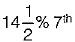harmonic.

QUESTION: 4

In a single-pulse modulation of PWM inverters if pulse width is 120° then

Solution:

The rms value of amplitude of harmonic voltage of a single, pulse modulated wave is given by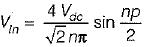(where, p = width of pulse an Vdc = supply dc voltage)
If the 3rd harmonic Is to be eliminated, then
EL3 = 0
i.e.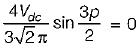or,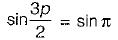or,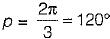= Required pulse width

QUESTION: 5

If for a single-phase half-bridge inverter, the amplitude of output voltage is V0 and the output power is P, then their corresponding values for a single-phase full-bridge inverter would be respectively

Solution: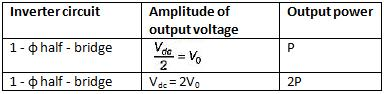QUESTION: 6

A single-phase full bridge inverter can operate in load-commutation mode in case load consists of

Solution:

In a 1-φ full bridge inverter if RLC load is underdamped, then the two thyristors (namely T1 and T2) shown in figure will get commutated naturally and therefore no commutation circuitry will be needed. Thus, load commutation will be possible.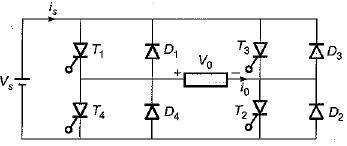QUESTION: 7

An inverter has a periodic output voltage with the output waveform as shown in figure.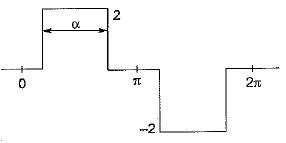When the conduction angle a = 120°, the rms value of the output voltage is

Solution: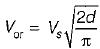(Here, 2 pulse width = 120°)
∴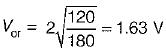QUESTION: 8

Assertion (A): The terminal voltage of a voltage source inverter remains substantially constant with variations in load.
Reason (R): Any short-circuit across the terminals of a voltage source inverter causes current to rise very fast.

Solution:

A voltage source inverter is one in which the d.c. source has small or negligible impedance. Due to low internal impedance, the terminal voltage of a VSI remains substantially constant with variations in load. Reason is also a correct -statement because due to low time constant of internal impedance any short-circuit across the terminals of a VSI causes current to rise very fast. Hence, both assertion and reason are true but reason is not the correct explanation of assertion.

QUESTION: 9

Assertion (A): The lower order harmonics are reduced by using some technique while the higher order harmonics are reduced by using filter.
Reason (R): The cost of the filter is reduced and at the same time transient response is also improved to a great extent.

Solution:
QUESTION: 10

Inverters designed from BJT are preferably used in saturation region than in active region because of

Solution:Use Code STAYHOME200 and get INR 200 additional OFF Use Coupon Code

Track your progress, build streaks, highlight & save important lessons and more!

Similar ContentRelated tests